# Cell Noise

### What is Cell Noise?

Cell noise (sometimes called Worley noise) is a is a procedural texture primitive like Perlin Noise. Despite its numerous applications there are few good implementations and explanations available online. Perhaps the best way to be introduced to cell noise  is with a demo. By the end of this blog post you will understand all of the options available in the demo 🙂

### Theory

The evaluated value of cell noise at a given position is simply the distance to the nearest feature point. Feature points are points placed randomly throughout space. The image below illustrates this.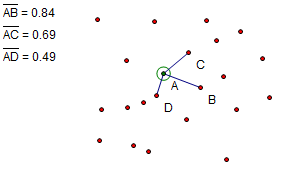In the above image the circled green dot (point A) is the position at which the noise function is being evaluated and all the red dots are the feature points.  Feature point D is the closest point to the evaluation point, A. The noise function evaluated at point A is the distance between point A and point D (0.49 in this case).

### Naive Implementation

The obvious implementation of cell noise is to:

1. Generate and store a bunch of random feature points
2. Evaluate a point by looking through all feature points and finding the closest point.

There are several major drawbacks to this naive implementation including storage requirements and computational cost making the naive implementation impractical. In 1996, Steven Worley devised an efficient algorithm for  evaluating cell noise. The rest of this blog post will detail his algorithm.

### Efficient Implementation

Evaluating a point using Steven Worley’s algorithm consist of the following steps

#### 1. Determine which cube the evaluation point is in

Steven Worley’s algorithm divides space into a regular grid of cubes whose locations are at integer locations. In the image below the evaluation point is the green dot, point A. Point A is located at ( 3.45, 2.65) in the cube ( 3, 2).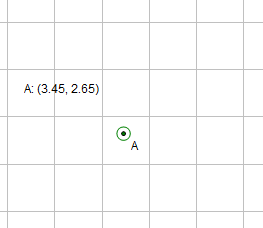#### 2. Generate a random number generator for the cube

We need to generate a reproducible random number generator uniquely seeded for the cube. It is critical that the seed is the same each time a point is evaluated within the cube. The seed can be created by hashing the integer coordinates of the cube. There are many hash functions suitable for this purpose. In the demo and sample code I used the FNV hashing algorithm. Once we have a seed unique to the cube we can generate random numbers using a LCG random number generator.

#### 3. Determine how many feature points are in the cube

In order to determine how many feature points are in the cube we must consider how feature points are distributed in space. The simplest distribution is probably the Poisson distribution, feature points are placed randomly and independently of each other. The only parameter the Poisson distribution requires is the average number of feature points per unit space. Using the discrete Poisson distribution function we can calculate the probability of having 0,1,2, or more points in a cube. By generating a random number between zero and one (using the generator from step 2) and comparing its value with the calculated probabilities we can determine the number of feature points in a specific cube. For performance reasons, which will be discuss later, the number of points in a cube will be clamped between one and nine.

#### 4. Randomly place the feature points in the cube

Using the random number generator initialized in step 2, we generate coordinates for each feature point are inserted in the cube.

#### 5. Find the feature point closest to the evaluation point

As we place the feature points into the cube we do not store them. Rather, we simply keep track of the distance to the closest feature point inserted thus far. When we are done placing all the feature points we have found the closest feature point.

#### 6. Check the neighboring cubes to ensure their are no closer evaluation points.

The closest feature point to the evaluation point may not be in the same cube as the evaluation point. The image below is an example of such a case.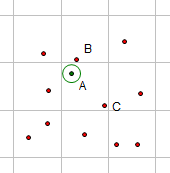In the above image the closest feature point to the evaluation point A is obviously the feature point B which is not in the same cube as the evaluation point A.

Because the closest feature point may not be in the same cube as the evaluation point all the neighboring cubes must be checked to see if they contain the closest feature point. This is done by repeating steps 2 through 5 for each neighboring cube.

In step 3 we clamped the number of feature points in a cube between 1 and 9. The lower bound of 1 guarantees that the closest feature point is either in the same cube as the evaluation point or in an immediately neighboring cube. If we do not have this lower bound we may have to check many neighbors and performance would suffer. In the image below each cube is not required to have at least one feature point. The closest feature point to the evaluation point A is feature point E. Feature point E is not in the same cube as the evaluation cube or in an immediately neighboring cube.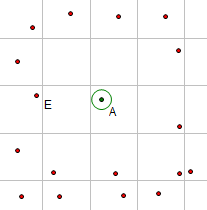### Extensions

#### Combining the N closest feature points

So far we have only concerned ourselves with finding the distance to the closest feature point. If we find the distances to the closest several feature points we can combine their distances to achieve some cool effects. For example, in the image below the value at the evaluation point is the distance to the second closest feature point minus the distance to the closest feature point.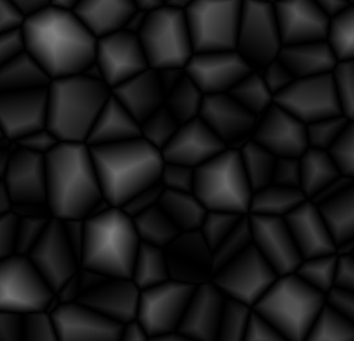Keeping track of the closest n feature points requires a simple modification to our algorithm. Instead of only keeping track of the distance to the closest point (for step 5), we keep track of an array of the distances to the closest n feature points. To determine if a feature point is in the closest n feature points we simply insert the feature point’s distance from the evaluation point into the array using insertion sort.

#### Alternative Distance Functions

To this point we have been measuring distance using the the euclidean distance metric. There are other distance metrics which will produce very different result. Look at the images below for examples of different distance metrics.

• Euclidean distance metric• Manhattan distance metric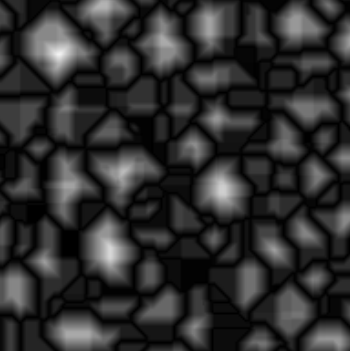• Chebyshev distance metric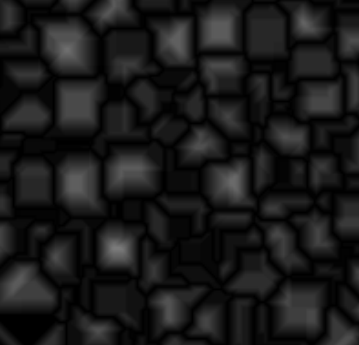### Code

The commented critical code from the demo is listed below. The full source for the demo is hosted at github and can be found at https://github.com/freethenation/CellNoiseDemo. The source code below is not optimized for performance but rather written to be easily understood. I may post an optimized version at some later time.

```public static class CellNoise
{
/// <summary>
/// Generates Cell Noise
/// </summary>
/// <param name="input">The location at which the cell noise function should be evaluated at.</param>
/// <param name="seed">The seed for the noise function</param>
/// <param name="distanceFunc">The function used to calculate the distance between two points. Several of these are defined as statics on the WorleyNoise class.</param>
/// <param name="distanceArray">An array which will store the distances computed by the Worley noise function.
/// The length of the array determines how many distances will be computed.</param>
/// <param name="combineDistancesFunc">The function used to color the location. The color takes the populated distanceArray
/// param and returns a float which is the greyscale value outputed by the worley noise function.</param>
/// <returns>The color worley noise returns at the input position</returns>
public static Vector4 CellNoiseFunc(this Vector3 input, int seed, Func<Vector3, Vector3, float> distanceFunc, ref float[] distanceArray, Func<float&#91;&#93;, float> combineDistancesFunc)
{
//Declare some values for later use
uint lastRandom, numberFeaturePoints;
Vector3 randomDiff, featurePoint;
int cubeX, cubeY, cubeZ;

//Initialize values in distance array to large values
for (int i = 0; i < distanceArray.Length; i++)
distanceArray&#91;i&#93; = 6666;

//1. Determine which cube the evaluation point is in
int evalCubeX = (int)Math.Floor(input.X);
int evalCubeY = (int)Math.Floor(input.Y);
int evalCubeZ = (int)Math.Floor(input.Z);

for (int i = -1; i < 2; ++i)
for (int j = -1; j < 2; ++j)
for (int k = -1; k < 2; ++k)
{
cubeX = evalCubeX + i;
cubeY = evalCubeY + j;
cubeZ = evalCubeZ + k;

//2. Generate a reproducible random number generator for the cube
lastRandom = lcgRandom(hash((uint)(cubeX + seed), (uint)(cubeY), (uint)(cubeZ)));
//3. Determine how many feature points are in the cube
numberFeaturePoints = probLookup(lastRandom);
//4. Randomly place the feature points in the cube
for (uint l = 0; l < numberFeaturePoints; ++l)
{
lastRandom = lcgRandom(lastRandom);
randomDiff.X = (float)lastRandom / 0x100000000;

lastRandom = lcgRandom(lastRandom);
randomDiff.Y = (float)lastRandom / 0x100000000;

lastRandom = lcgRandom(lastRandom);
randomDiff.Z = (float)lastRandom / 0x100000000;

featurePoint = new Vector3(randomDiff.X + (float)cubeX, randomDiff.Y + (float)cubeY, randomDiff.Z + (float)cubeZ);

//5. Find the feature point closest to the evaluation point.
//This is done by inserting the distances to the feature points into a sorted list
insert(distanceArray, distanceFunc(input, featurePoint));
}
//6. Check the neighboring cubes to ensure their are no closer evaluation points.
// This is done by repeating steps 1 through 5 above for each neighboring cube
}

float color = combineDistancesFunc(distanceArray);
if(color < 0) color = 0;
if(color > 1) color = 1;
return new Vector4(color, color, color, 1);
}

public static float EuclidianDistanceFunc(Vector3 p1, Vector3 p2)
{
return (p1.X - p2.X) * (p1.X - p2.X) + (p1.Y - p2.Y) * (p1.Y - p2.Y) + (p1.Z - p2.Z) * (p1.Z - p2.Z);
}

public static float ManhattanDistanceFunc(Vector3 p1, Vector3 p2)
{
return Math.Abs(p1.X - p2.X) + Math.Abs(p1.Y - p2.Y) + Math.Abs(p1.Z - p2.Z);
}

public static float ChebyshevDistanceFunc(Vector3 p1, Vector3 p2)
{
Vector3 diff = p1 - p2;
return Math.Max(Math.Max(Math.Abs(diff.X), Math.Abs(diff.Y)), Math.Abs(diff.Z));
}

/// <summary>
/// Given a uniformly distributed random number this function returns the number of feature points in a given cube.
/// </summary>
/// <param name="value">a uniformly distributed random number</param>
/// <returns>The number of feature points in a cube.</returns>
// Generated using mathmatica with "AccountingForm[N[Table[CDF[PoissonDistribution, i], {i, 1, 9}], 20]*2^32]"
private static uint probLookup(uint value)
{
if (value < 393325350) return 1;
if (value < 1022645910) return 2;
if (value < 1861739990) return 3;
if (value < 2700834071) return 4;
if (value < 3372109335) return 5;
if (value < 3819626178) return 6;
if (value < 4075350088) return 7;
if (value < 4203212043) return 8;
return 9;
}

/// <summary>
/// Inserts value into array using insertion sort. If the value is greater than the largest value in the array
/// it will not be added to the array.
/// </summary>
/// <param name="arr">The array to insert the value into.</param>
/// <param name="value">The value to insert into the array.</param>
private static void insert(float[] arr, float value)
{
float temp;
for (int i = arr.Length - 1; i >= 0; i--)
{
if (value > arr[i]) break;
temp = arr[i];
arr[i] = value;
if (i + 1 < arr.Length) arr&#91;i + 1&#93; = temp;
}
}

/// <summary>
/// LCG Random Number Generator.
/// LCG: http://en.wikipedia.org/wiki/Linear_congruential_generator
/// </summary>
/// <param name="lastValue">The last value calculated by the lcg or a seed</param>
/// <returns>A new random number</returns>
private static uint lcgRandom(uint lastValue)
{
return (uint)((1103515245u * lastValue + 12345u) % 0x100000000u);
}

/// <summary>
/// Constant used in FNV hash function.
/// FNV hash: http://isthe.com/chongo/tech/comp/fnv/#FNV-source
/// </summary>
private const uint OFFSET_BASIS = 2166136261;
/// <summary>
/// Constant used in FNV hash function
/// FNV hash: http://isthe.com/chongo/tech/comp/fnv/#FNV-source
/// </summary>
private const uint FNV_PRIME = 16777619;
/// <summary>
/// Hashes three integers into a single integer using FNV hash.
/// FNV hash: http://isthe.com/chongo/tech/comp/fnv/#FNV-source
/// </summary>
/// <returns>hash value</returns>
private static uint hash(uint i, uint j, uint k)
{
return (uint)((((((OFFSET_BASIS ^ (uint)i) * FNV_PRIME) ^ (uint)j) * FNV_PRIME) ^ (uint)k) * FNV_PRIME);
}
}
```

### Conclusion

I hope this post has provided you with an understanding of what cell noise is and how it can be efficiently implemented. If you are confused about anything above leave a comment or read Steven Worley’s paper on cell noise.